## Designing of islamic arabesques

by: Maple

Elena, Liya "Designing of islamic arabesques", Kazan, Russia, school #57

> restart:
At the theorem of cosines  c^2 = a^2+b^2-2*a*b*cos(phi);
In our case  c=a0 ,  a=1 ,  a=b , phi; - acute angle of a rhombus (the tip of the kalam).
s0 calculated at theorem of  Pythagoras.
(а0 - horizontal diagonal of a  rhombus, s0 - vertical diagonal of a  rhombus)
> a:=1:phi:=Pi/4:
> a0:=sqrt(a^2+a^2-2*a^2*cos(phi));

a0 := sqrt(2 - sqrt(2))

> solve((s0^2)/4=a^2-(a0^2)/4,s0);

sqrt(2 + sqrt(2)), -sqrt(2 + sqrt(2))

The setting of initial parameters : the size of the tip of the pen-kalam and  depending on its - the main module size - point
(а0 - horizontal diagonal of a  rhombus, s0 - vertical diagonal of a  rhombus)
> a0:=sqrt(2-sqrt(2)):
> s0:=sqrt(2+sqrt(2)):
Connection the graphical libraries Maple
> with(plots):with(plottools):
Construction of unit of measure (point) - rhombus - the tip of the kalam
> p0:=plot([[0,0],[a0/2,s0/2],[0,s0],[-a0/2,s0/2],[0,0]],scaling=constrained,color=gold,thickness=3):
> plots[display](p0);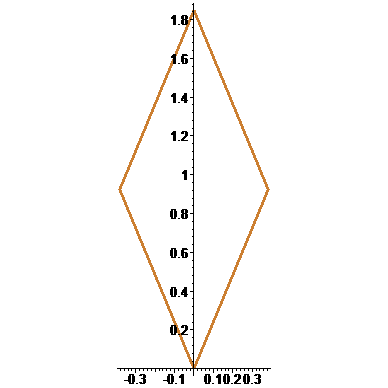The setting and construction of altitude of alif - the basis of the rules compilation of the proportions      Example, on style naskh altitude of alif amount five points
> p_i:=seq(plot([[0,0+s0*i],[a0/2,s0/2+s0*i],[0,s0+s0*i],[-a0/2,s0/2+s0*i],[0,0+s0*i]],scaling=constrained,color=black),i=0..4):
> pi:=display(p_i):
> plots[display](p_i);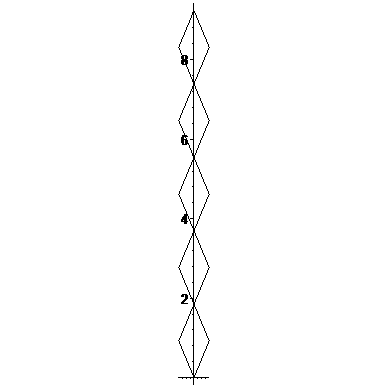The setting of appropriate circle of diameter, amount altitude of alifd0:=s0+s0*i:
> i:=4:
> d0:=d0:
> c0:=circle([0,d0/2],d0/2,color=blue):
> plots[display](p_i,c0);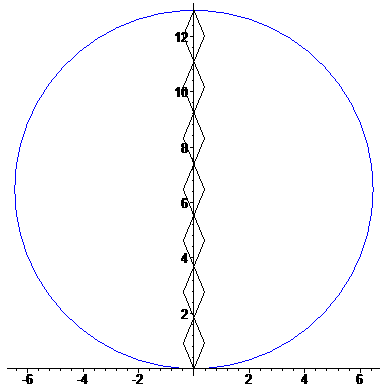Construction of flower by turning "point"r_i:=seq(rotate(p0,i*Pi/4),i=1..8):
> p1:=display(r_i,p0):plots[display](p1,scaling=constrained);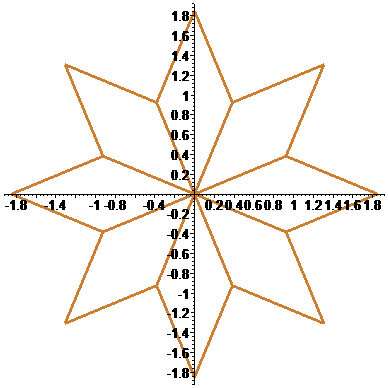The setting of circumscribed circlec1:=circle([0,0],s0,color=blue,thickness=2):
Construction and the setting of flower inscribed in a circle
> plots[display](c1,p1,scaling=constrained);
> p_c:=plots[display](c1,p1,scaling=constrained):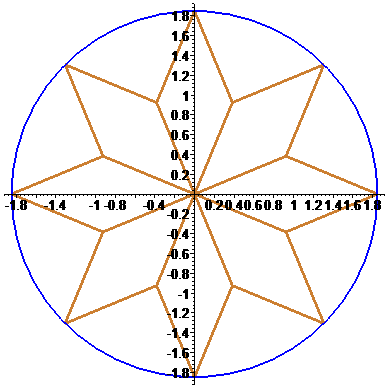The setting and construction of arabesque by horizontal parallel transport original flower with different stepspt_i_1:=seq(translate(p1,5*a0*i,0),i=0..4):
> plots[display](pt_i_1);
> pt_i_11:=seq(translate(p1,2*a0*i,0),i=0..4):
> plots[display](pt_i_11);
> pt_i_111:=seq(translate(p1,a0*7*i,0),i=0..4):
> plots[display](pt_i_111);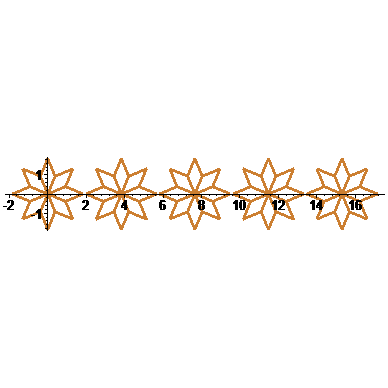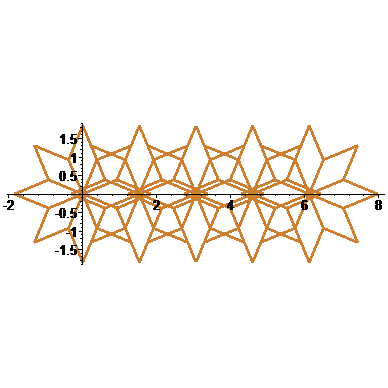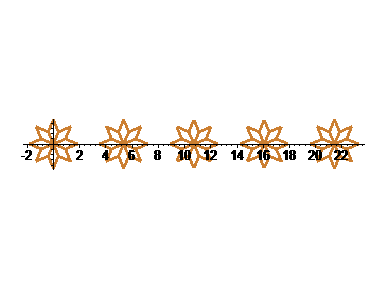The setting and construction of arabesque by vertical parallel transport original flower with different stepspt_i_2:=seq(translate(p1,0,2*s0*i),i=0..4):
> plots[display](pt_i_2);
> pt_i_22:=seq(translate(p1,0,s0*i),i=0..4):
> plots[display](pt_i_22);
> pt_i_222:=seq(translate(p1,0,1/2*s0*i),i=0..4):
> plots[display](pt_i_222);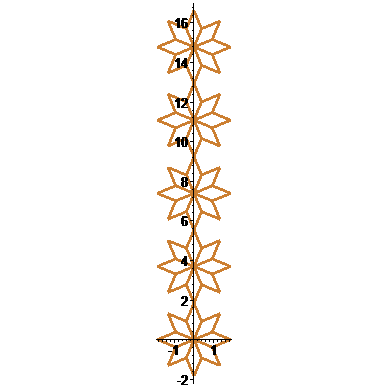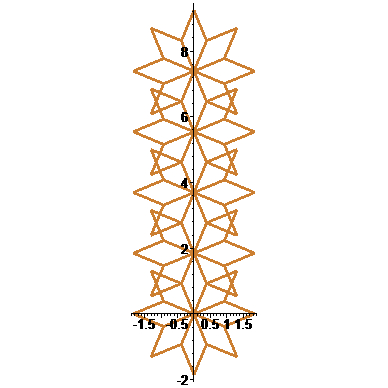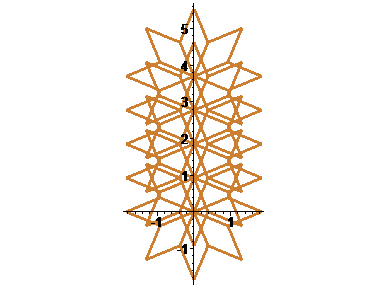Getting arabesques by turning original flower on different anglespr:=rotate(p1,Pi/8):
> plots[display](pr);
> plots[display](p1,pr);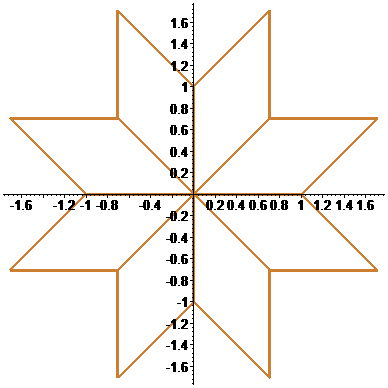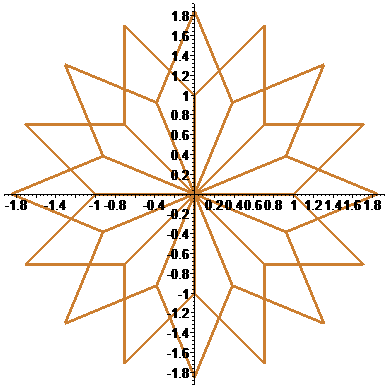> pr_i:=seq(rotate(p1,Pi/16*i),i=0..8):
> plots[display](pr_i);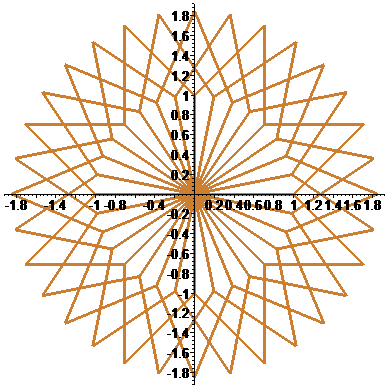> pt_1:=translate(p1,0,2*s0):
> pr_1_i:=seq(rotate(pt_1,Pi/3.5*i),i=0..6):
> plots[display](pr_1_i);
> pr_11_i:=seq(rotate(pt_1,Pi/5*i),i=0..10):
> plots[display](pr_11_i);
> pr_111_i:=seq(rotate(pt_1,Pi/6.5*i),i=0..12):
> plots[display](pr_111_i);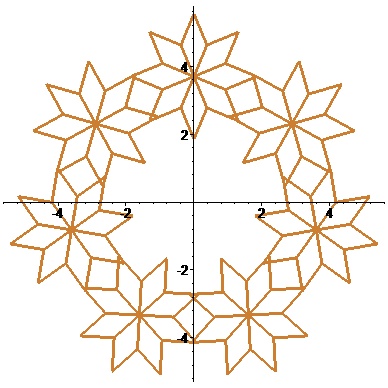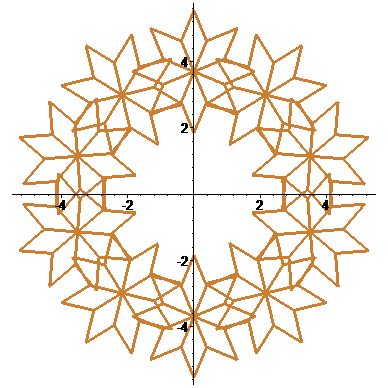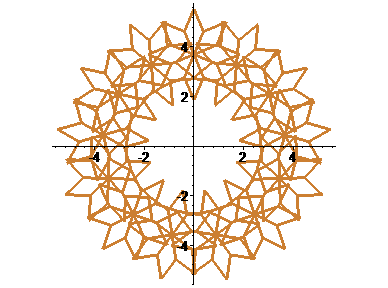by: Maple

Muchametshina Liya,  8th class,  school № 57, Kazan, Russia

Square

Rectangle

Rhombus

Parallelogram

Trapeze

Construction of square

> restart:
> with(plottools):
Сoordinates (x;y) of the lower left corner of the square and the side "а"
> x:=0;y:=3;a:=6;

x := 0

y := 3

a := 6

Construction of the square
> P1:=plot([[x,y],[x,y+a],[x+a,y+a],[x+a,y],[x,y]],color=green,thickness=4):
> plots[display](P1,scaling=CONSTRAINED);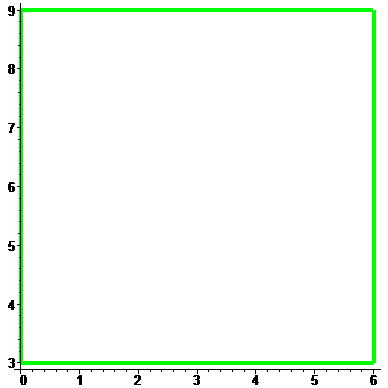The setting of the second square wich moved relative to the first on the vector (2;-3) (vector can be changed) and with side "а-1" (the length of a side can be changed)P2:=plot([[x+2,y-3],[x+2,y-3+a-1],[x+2+a-1,y-3+a-1],[x+2+a-1,y-3],[x+2,y-3]],color=black,thickness=4):
> plots[display](P1,P2,scaling=CONSTRAINED);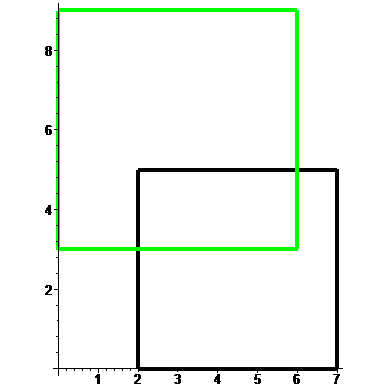Construction of rectangle

> restart:
> with(plottools):
Сoordinates (x;y) of the lower left corner of the square and the "а" and "b" sides
> x:=0;y:=2;a:=3;b:=9;
>

x := 0

y := 2

a := 3

b := 9

The rectangle is specified by the sequence of vertices with given the lengths "a" and "b"
> l:=plot([[x,y],[x,y+a],[x+b,y+a],[x+b,y],[x,y]]):
> plots[display](l,scaling=CONSTRAINED,thickness=4);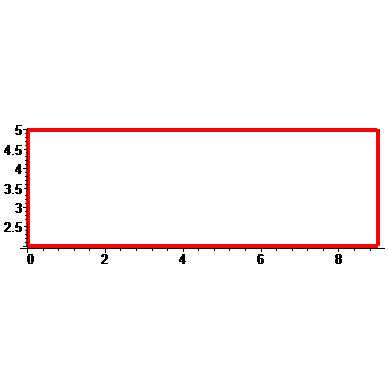Construction of rhombus

> restart:
> with(plottools):
The coordinates (x;y) of the initial vertex of the rhombus and the half of the diagonals "a" and "b"
> x:=0;y:=2;a:=3;b:=4;

x := 0

y := 2

a := 3

b := 4

Rhombus is specified by the sequence of vertices with the values "a" and "b"
> ll:=plot([[x,y],[x+a,y+b],[x+a+a,y],[x+a,y-b],[x,y]]):
> plots[display](ll,scaling=CONSTRAINED,thickness=4);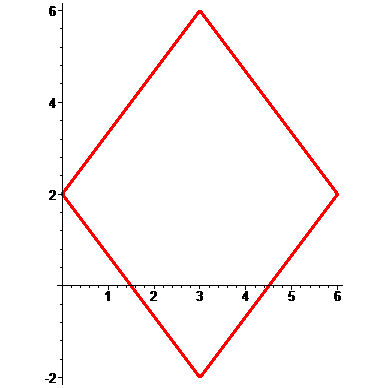Construction of parallelogram

> restart:
> with(plottools):
(х;у) - the starting point, (i;j) - the displacement vector of starting point, "а" - the base of the parallelogram
> x:=0;y:=0;i:=4;j:=5;a:=10;

x := 0

y := 0

i := 4

j := 5

a := 10

The parallelogram is defined by the sequence of vertices
> P1:=plot([[x,y],[x+i,y+j],[x+i+a,y+j],[x+a,y],[x,y]]):
> plots[display](P1,scaling=CONSTRAINED,thickness=4);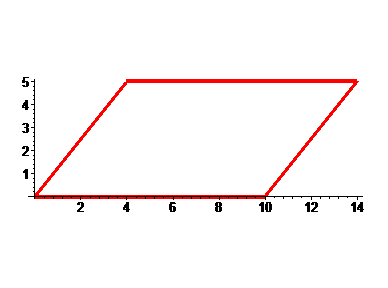If  i= 0  it turns out the rectangleget.
If  j= а  it turns out the  square.
If  a := sqrt(i^2+j^2) it turns out the rhombus. a:=sqrt(i^2+j^2):

Construction of trapeze

Trapeze general form
> restart:
> with(plottools):
>
(х;у) - the starting point, (i;j) - the displacement vector of starting point, а - the larger base of the trapezoid
> x:=0;y:=2;i:=1;j:=5;a:=11;

x := 0

y := 2

i := 1

j := 5

a := 11

The trapez is defined by the sequence of vertices
> P1:=plot([[x,y],[x+i,y+j],[x+i+j,y+j],[x+i+a,y],[x,y]]):
> plots[display](P1,scaling=CONSTRAINED,thickness=4);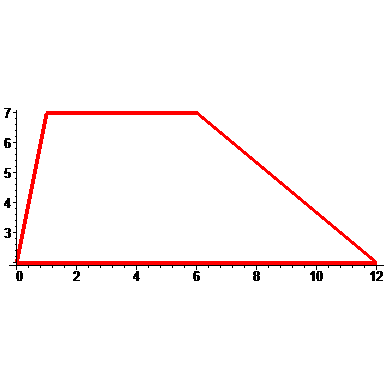Rectangular trapezoid
> restsrt:
> with(plottools):
> x:=0;y:=2;i:=0;j:=6;a:=11;

x := 0

y := 2

i := 0

j := 6

a := 11

> P1:=plot([[x,y],[x,y+j],[x+j,y+j],[x+a,y]]):
> plots[display](P1,scaling=CONSTRAINED,thickness=4);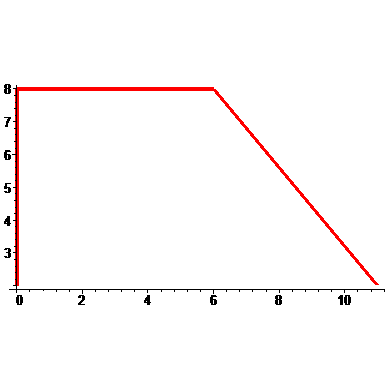Isosceles trapezoid
> restart:
> with(plottools):
> x:=0;y:=2;i:=4;j:=6;a:=15;

x := 0

y := 2

i := 4

j := 6

a := 15

> P1:=plot([[x,y],[x+i,y+j],[x+j+i,y+j],[x+a,y],[x,y]]):
> plots[display](P1,scaling=CONSTRAINED,thickness=4);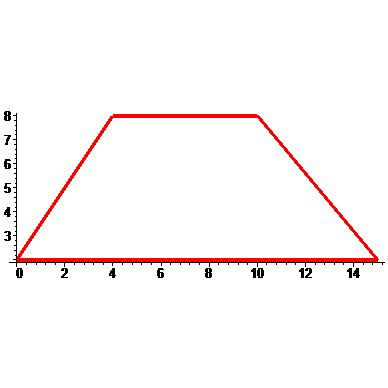## Eurocup simulation in Maple

Anyone interested in modifying and expanding the Maple FIFA simulation for the Eurocup to include the knockout round?  Any interest?

## Gialid_Plots_transformation

by: Maple , MaplePrimes

> restart;
> a := -10; b := 10; ps := seq(plot([i, t, t = -20 .. 20], x = -10 .. 10, y = -20 .. 20, color = red, style = point), i = a .. b);

plots[display](ps, insequence = true); p := plots[display](ps, insequence = true);restart:
with(plots):
y=sin(x);
p:=implicitplot(y=sin(x),x=-10..10,y=-2..2,thickness=4,color=red,scaling=constrained,numpoints=1000):
plots[display](p);y=sin(3*x);
p0:=implicitplot(y=sin(x),x=-10..10,y=-5..5,thickness=3,color=red,scaling=constrained,numpoints=1000,linestyle=2,style=POINT,symbol=CROSS):
p1:=implicitplot(y=sin(3*x),x=-10..10,y=-5..5,thickness=4,color=blue,numpoints=10000):
plots[display](p0,p1);
y=sin(1/3*x);
p11:=implicitplot(y=sin(1/3*x),x=-10..10,y=-5..5,thickness=4,color=navy,numpoints=10000):
plots[display](p0,p11);y=2*sin(x);
p2:=implicitplot(y=2*sin(x),x=-10..10,y=-5..5,thickness=4,color=blue,numpoints=10000):
plots[display](p0,p2);
y=1/2*sin(x);
p22:=implicitplot(y=1/2*sin(x),x=-10..10,y=-5..5,thickness=4,color=navy,numpoints=10000):
plots[display](p0,p22);y=2+sin(x);
p3:=implicitplot(y=2+sin(x),x=-10..10,y=-5..5,thickness=4,color=blue,numpoints=10000):
plots[display](p0,p3);
y=sin(x)-2;
p33:=implicitplot(y=sin(x)-2,x=-10..10,y=-5..5,thickness=4,color=navy,numpoints=10000):
plots[display](p0,p33);y=sin(x+2);
p4:=implicitplot(y=sin(x+2),x=-10..10,y=-5..5,thickness=4,color=blue,numpoints=10000):
plots[display](p0,p4);
y=sin(x-2);
p44:=implicitplot(y=sin(x-2),x=-10..10,y=-5..5,thickness=4,color=navy,numpoints=10000):
plots[display](p0,p44);y=-sin(x);
p7:=implicitplot(y=-sin(x),x=-10..10,y=-5..5,thickness=4,color=blue,numpoints=10000):
plots[display](p0,p7);
y=sin(-x);
p77:=implicitplot(y=sin(-x),x=-10..10,y=-5..5,thickness=4,color=navy,numpoints=10000):
plots[display](p0,p77);y=abs(sin(x));
p00:=implicitplot(y=sin(x),x=-10..10,y=-5..5,thickness=3,color=red,scaling=constrained,numpoints=1000,linestyle=2,style=POINT,symbol=BOX):
p5:=implicitplot(y=abs(sin(x)),x=-10..10,y=-5..5,thickness=4,color=blue,numpoints=10000):
plots[display](p00,p5);
plots[display](p5,scaling=constrained);y=sin(abs(x));
p00:=implicitplot(y=sin(x),x=-10..10,y=-5..5,thickness=3,color=red,scaling=constrained,numpoints=1000,linestyle=2,style=POINT,symbol=BOX):
p6:=implicitplot(y=sin(abs(x)),x=-10..10,y=-5..5,thickness=4,color=navy,numpoints=10000):
plots[display](p00,p6);
plots[display](p6,scaling=constrained);## Russian secondary school Maple project

by: Maple

Post gialid_GEODROMchik - what is this?

Pilot project of Secondary school # 57 of Kazan, Russia

Use of Maple

in Mathematics Education by mathematics teacher Alsu Gibadullina

and in scientific work of schoolchildren

the 6th class

Arina                         Elza                             DavidArtur## A New "Section" Style with Programmatic Table...

by: Maple 2016

A few people have asked me how I created the sections in the Maple application in this video: https://youtu.be/voohdmfTRn0?t=572

Here's the worksheet (Maple 2016 only). As you can see, the “sections” look different what you would normally expect (I often like to experiment with small changes in presentation!)These aren't, however, sections in the traditional Maple sense; they're a demonstration of Maple 2016's new tools for programmatically changing the properties of a table (including the visibility of its rows and columns). @dskoog gets the credit for showing me the technique.

Each "section" consists of a table with two rows.

• The table has a name, specified in its properties.
• The first row (colored blue) contains (1) a toggle button and (2) the title of each section (with the text in white)
• The second row (colored white) is visible or invisible based upon the state of the toggle button, and contains the content of my section.

Each toggle button has

• a name, specified in its properties
• + and - images associated with its on and off states (with the image background color matching the color of the first table row)
• Click action code that enables or disables the visibility of the second row

The Click action code for the toggle button in the "Pure Fluid Properties" section is, for example,

tableName:="PureFluidProperties_tb":
buttonName:="PureFluidProperties_tbt":
if DocumentTools:-GetProperty(buttonName, 'value') = "false" then
DocumentTools:-SetProperty([tableName, 'visible[2..]', true]);
else
DocumentTools:-SetProperty([tableName, 'visible[2..]', false]);
end if;

As I said at the start, I often try to make worksheets look different to the out-of-the-box defaults. Programmatic table properties have simply given me one more option to play about with.

## Can Maple T.A. be a 'tolerant' marker?

by:Disclaimer: This blog post has been contributed by Prof. Nicola Wilkin, Head of Teaching Innovation (Science), College of Engineering and Physical Sciences and Jonathan Watkins from the University of Birmingham Maple T.A. user group*.

Written for Maple T.A. 2016. For Maple T.A. 10 users, this question can be written using the queston designer.## An Interactive Application for Exploring Country...

by: Maple 2015

This is the second of three blog posts about working with data sets in Maple.

In my previous post, I discussed how to use Maple to access a large number of data sets from Quandl, an online data aggregator. In this post, I’ll focus on exploring built-in data sets in Maple.

Data is being generated at an ever increasing rate. New data is generated every minute, adding to an expanding network of online information. Navigating through this information can be daunting. Simply preparing a tabular data set that collects information from several sources is often a difficult and time consuming effort. For example, even though the example in my previous post only required a couple of lines of Maple code to merge 540 different data sets from various sources, the effort to manually search for and select sources for data took significantly more time.

In an attempt to make the process of finding data easier, Maple’s built-in country data set collects information on country-specific variables including financial and economic data, as well as information on country codes, population, area, and more.

The built-in database for Country data can be accessed programmatically by creating a new DataSets Reference:

`CountryData := DataSets:-Reference( "builtin", "country" );`This returns a Reference object, which can be further interrogated. There are several commands that are applicable to a DataSets Reference, including the following exports for the Reference object:

`exports( CountryData, static );`

The list of available countries in this data set is given using the following:

`GetElementNames( CountryData );`The available data for each of these countries can be found using:

`GetHeaders( CountryData );`There are many different data sets available for country data, 126 different variables to be exact. Similar to Maple’s DataFrame, the columns of information in the built-in data set can be accessed used the labelled name.

For example, the three-letter country codes for each country can be returned using:

`CountryData[.., "3 Letter Country Code"];`

The three-letter country code for Denmark is:

`CountryData["Denmark", "3 Letter Country Code"];`Built-in data can also be queried in a similar manner to DataFrames. For example, to return the countries with a population density less than 3%:

`pop_density := CountryData[ .., "Population Density" ]:`
`pop_density[ `Population Density` < 3 ];`At this time, Maple’s built-in country data collection contains 126 data sets for 185 countries. When I built the example from my first post, I knew exactly the data sets that I wanted to use and I built a script to collect these into a larger data container. Attempting a similar task using Maple’s built-in data left me with the difficult decision of choosing which data sets to use in my next example.

So rather than choose between these available options, I built a user interface that lets you quickly browse through all of Maple’s collection of built-in data.Using a couple of tricks that I found in the pages for Programmatic Content Generation, I built the interface pictured above. (I’ll give more details on the method that I used to construct the interface in my next post.)

This interface allows you to select from a list of countries, and visualize up to three variables of the country data with a BubblePlot. Using the preassigned defaults, you can select several countries and then visualize how their overall number of internet users has changed along with their gross domestic product. The BubblePlot visualization also adds a third dimension of information by adjusting the bubble size according to the relative population compared with the other selected countries.

Now you may notice that the list of available data sets is longer than the list of available options in each of the selection boxes. In order to be able to generate BubblePlot animations, I made an arbitrary choice to filter out any of the built-in data sets that were not of type TimeSeries. This is something that could easily be changed in the code. The choice of a BubblePlot could also be updated to be any other type of Statistical visualization with some additional modifications.

You can also interact with it via the MapleCloud: http://maplecloud.maplesoft.com/application.jsp?appId=5743882790764544

I’ll be following up this post with an in-depth post on how I authored the country selector interface using programmatic content generation.

## Quantum Entanglement and Maple

by: Maple

Since it's not every day we receive submission to the Maple Application Center that have words like "quantum entanglement" (and "teleportation"!) in the title, I thought I'd share this one:

Matrix Representation of Quantum Entangled States: Understanding Bell's Inequality and Teleportation

eithne

## Document vs worksheet mode

by: Maple

I'm an educator (physicist) who has migrated to Maple because of the lower "activation barrier" to get something of interest produced by the student. The students in my courses are exposed to several language (Python, C++, Java) and mathematical systems (Mathematica, Maple, MATLAB.) Many claim that unless forced to used a particular language or system, their first choice is Python and Maple for the reason I cite.

As a consequence, it is my experience that students truly perfer the math-like appearance of the 2-D Math notation as opposed to the Maple notation. They see it as more natural - again with a lower activation barrier. Hence I see no reason to change. However, I would be interested in reasons why it might be beneficial.

My ultimate question is: do I start them with worksheet mode or documents mode? I'm use to worksheet mode and have found the call and response method easy for them to understand. But document mode has many valuable benefits. Is it worth the increase in learning (and frustration) for the benefits if the students use the software only a few times per semester? Or for some, every week?

I would be interested in hearing about the experiences of other educators.

## From combinatorics: an algorithm to enumerate...

by: Maple

Greetings to all. I am writing today to share a personal story / exploration using Maple of an algorithm from the history of combinatorics. The problem here is to count the number of strings over a certain alphabet which consist of some number of letters and avoid a set of patterns (these patterns are strings as opposed to regular expressions.) This counting operation is carried out using rational generating functions that encode the number of admissible strings of length n in the coefficients of their series expansions. The modern approach to this problem uses the Goulden-Jackson method which is discussed, including a landmark Maple implementation from a paper by D. Zeilberger and J. Noonan, at the following link at math.stackexchange.com (Goulden-Jackson has its own website, all the remaining software described in the following discussion is available at the MSE link.) The motivation for this work was a question at the MSE link about the number of strings over a two-letter alphabet that avoid the pattern ABBA.

As far as I know before Goulden-Jackson was invented there was the DFA-Method (Deterministic Finite Automaton also known as FSM, Finite State Machine.) My goal in this contribution was to study and implement this algorithm in order to gain insight about its features and how it influenced its powerful successor. It goes as follows for the case of a single pattern string: compute a DFA whose states represent the longest prefix of the pattern seen at the current position in the string as it is being scanned by the DFA, with the state for the complete pattern doubling as a final absorbing state, since the pattern has been seen. Translate the transitions of the DFA into a system of equations in the generating functions representing strings ending with a given maximal prefix of the pattern, very much like Markov chains. Finally solve the system of equations for the generating functions and thus obtain the sequence of values of strings of length n over the given alphabet that avoid the given pattern.

I have also implemented the DFA method for sets of patterns as opposed to just one pattern. The algorithm is the same except that the DFA does not consist of a chain with backlinks as in the case of a single pattern but a tree of prefixes with backlinks to nodes higher up in the tree. The nodes in the tree represent all prefixes that need to be tracked where obviously a common prefix between two or more patterns is shared i.e. only represented once. The DFA transitions emanating from nodes that are leaves represent absorbing states indicating that one of the patterns has been seen. We run this algorithm once it has been verified that the set of patterns does not contain pairs of patterns where one pattern is contained in another, which causes the longer pattern to be eliminated at the start. (Obviously if the shorter pattern is forbidden the so is the longer.) The number of states of the DFA here is bounded above by the sum of the lengths of the patterns with subpatterns eliminated. The uniqueness property of shared common prefixes holds for subtrees of the main tree i.e. recursively. (The DFA method also copes easily with patterns that have to occur in a certain order.)

I believe the Maple code that I provide here showcases many useful tricks and techniques and can help the reader advance in their Maple studies, which is why I am alerting you to the web link at MSE. I have deliberately aimed to keep it compatible with older versions of Maple as many of these are still in use in various places. The algorithm really showcases the power of Maple in combinatorics computing and exploits many different aspects of the software from the solution of systems of equations in rational generating functions to the implementation of data structures from computer science like trees. Did you know that Maple permits nested procedures as known to those who have met Lisp and Scheme during their studies? The program also illustrates the use of unit testing to detect newly introduced flaws in the code as it evolves in the software life cycle.

Enjoy and may your Maple skills profit from the experience!

Best regards,

Marko Riedel

The software is also available here: dfam-mult.txt

## Equidistant surface

by: Maple 15

Example of the equidistant surface at a distance of 0.25 to the surface
x3
-0.1 * (sin (4 * x1) + sin (3 * x2 + x3) + sin (2 * x2)) = 0
Constructed on the basis of universal parameterization of surfaces.

equidistant_surface.mw## Numerov algorithm in Maple

Maple 18

Hi there, fellow primers, it's good to be back after almost 5 years! I just want to share a worksheet on Numerov's algorithm in Maple using procedures as I've recently found out that google could not find any Maple procedure that implements Numerov's algorithm to solve ODEs.   numerov.mw   Reference.pdf

## Visualizing Multiple Quandl Data Sets using Bubble...

by: Maple 2015

This is the first of three blog posts about working with data sets in Maple.

In 2013, I wrote a library for Maple that used the HTTP package to access the Quandl data API and import data sets into Maple. I was motivated by the fact that, when I was downloading data, I often used multiple data sources, manually updated data when updates were available, and cleaned or manipulated the data into a standardized form (which left me spending too much time on the data acquisition step).

Simply put, I needed a source for data that would provide me with a searchable, stable data API, which would also return data in a form that did not require too much post-processing.

My initial library had really just scratched the surface of what was possible.

Maple 2015 introduced the new DataSets package, which fully integrated a data set search into core library routines and made its functionality more discoverable through availability in Maple’s search bar.

Accessing online data suddenly became much easier. From within Maple, I could now search through over 12 million time series data sets provided by Quandl, and then automatically import the data into a format that I could readily work with.

If you’re not already aware of this online service, Quandl is an online data aggregator that delivers a wide variety of high quality financial and economic data. This includes the latest data on stocks and commodities, exchange rates, and macroeconomic indicators such as population, inflation, unemployment, and so on. Quandl collects both open and proprietary data sets from many sources, such as the US Federal Reserve System, OECD, Eurostat, The World Bank, and Open Data for Africa. Best of all, Quandl's powerful API is free to use.One of the first examples for the DataSets package that I constructed was in part based on the inspirational work of Hans Rosling. I was drawn in by his ability to use statistical visualizations to break down complex multidimensional data sets and provide insight into underlying patterns; a key example investigating the correlation between rising incomes and life expectancy.

As well as online data, the DataSets package had a database for country data. Hence it seemed fitting to add an example that explored macroeconomic indicators for several countries. Accordingly, I set out to create an example that visualized variables such as Gross Domestic Product, Life Expectancy, and Population for a collection of countries.

I’ll now describe how I constructed this application.

The three key variables are Gross Domestic Product at Power Purchasing Parity, Life Expectancy, and Population. Having browsed through Quandl’s website for available data sets, the World Bank and Open Data for Africa projects seemingly had the most available relevant data; therefore I chose these as my data sources.

Pulling data for a single country from one of these sources was pretty straight forward. For example, the DataSets Reference for the Open Data for Africa data set on GDP at PPP for Canada is:

`DataSets:-Reference("quandl", "ODA/CAN_PPPPC"));`

In this command, the second argument is the Quandl data set code. If you are on Quandl’s website, this is listed near the top of the data set page as well as in the last few characters of the web address itself: https://www.quandl.com/data/ODA/CAN_PPPPC . Deconstructing the code, “ODA” stands for Open Data for Africa and the rest of the string is constructed from the three letter country code for Canada, “CAN”, and the code for the GDP and PPP. Looking at a small sample of other data set codes, I theorized that both of the data sources used a standardized data set name that included the ISO-3166 3-letter country code for available data sets. Based on this theory, I created a simple script to query for available data and discovered that there was data available for many countries using this standardized code. However, not every country had available data, so I needed to filter my list somewhat in order to pick only those countries for which information was available.

The script that I had constructed required three letter country codes. In order to test all available countries, I created a table to house the country names and three-letter country codes using data from the built-in database for countries:

`ccdata := DataSets:-Builtin:-Reference("country")[.., "3 Letter Country Code"];`
`cctable := table([seq(op(GetElementNames(ccdata[i])) = ccdata[i, "3 Letter Country Code"],                                 i = 1 .. CountRows(ccdata))]):`

My script filtered this table, returning a subset of the original table, something like:

`Countries := table( [“Canada” = “CAN”, “Sweden” = “SWE”, … ] );`

You can see the filtered country list in the code edit region of the application below.

With this shorter list of countries, I was now ready to download some data. I created three vectors to hold the data sets by mapping in the DataSets Reference onto the “standardized” data set names that I pulled from Quandl. Here’s the first vector for the data on GDP at PPP.

`V1 := Vector( [ (x) -> Reference("quandl", cat("ODA/", x, "_PPPPC"))`
`                   ~([entries(Countries, nolist, indexorder)])]):`
`#Open Data for Africa GDP at PPP`

Having created three data vectors consisting of 180 x 3 = 540 data sets, I was finally ready to visualize the large set of data that I had amassed.

In Maple’s Statistics package, BubblePlots can use the horizontal axis, vertical axis and the relative bubble size to illustrate multidimensional information. Moreover, if incoming data is stored as a TimeSeries object, BubblePlots can generate animations over a common period of time.

Putting all of this together generated the following animation for 180 available countries.This example will be included with the next version of Maple, but for now, you can download a copy here:DataSetsBubblePlot.mw

*Note: if you try this application at home, it will download 540 data sets. This operation plus the additional BubblePlot construction can take some time, so if you just want to see the finished product, you can simply interact with the animation in the Maple worksheet using the animation toolbar.

A more advanced example that uses multiple threads for data download can be seen at the bottom of the following page: https://www.maplesoft.com/products/maple/new_features/maple19/datasets_maple2015.pdf You can also interact with this example in Maple by searching for: ?updates,Maple2015,DataSets

In my next post, I’ll discuss how I used programmatic content generation to construct an interactive application for data retrieval.

## Bug in WhittakerW integration

Maple 2016Bug_in_integrate.mw

M_Iwaniuk

﻿